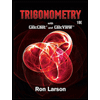Question

In Exercises 33–34, find the distance from the point to the line.

33. (0, 0, 12); x = 4t, y =-2t, z = 2t

34. (0, 0, 0); x = 5 + 3t, y = 5 + 4t, z =-3 - 5t

Expert Solution

### Want to see the full answer?

Check out a sample Q&A hereStudents who’ve seen this question also like:Trigonometry (MindTap Course List)
10th Edition
ISBN: 9781337278461
Author: Ron Larson
Publisher: Cengage Learning